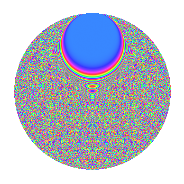# Properties

 Label 668.2.hLevel $668$ Weight $2$ Character orbit 668.h Rep. character $\chi_{668}(15,\cdot)$ Character field $\Q(\zeta_{166})$ Dimension $6724$ Newform subspaces $1$ Sturm bound $168$ Trace bound $0$

# Related objects

## Defining parameters

 Level: $$N$$ $$=$$ $$668 = 2^{2} \cdot 167$$ Weight: $$k$$ $$=$$ $$2$$ Character orbit: $$[\chi]$$ $$=$$ 668.h (of order $$166$$ and degree $$82$$) Character conductor: $$\operatorname{cond}(\chi)$$ $$=$$ $$668$$ Character field: $$\Q(\zeta_{166})$$ Newform subspaces: $$1$$ Sturm bound: $$168$$ Trace bound: $$0$$

## Dimensions

The following table gives the dimensions of various subspaces of $$M_{2}(668, [\chi])$$.

Total New Old
Modular forms 7052 7052 0
Cusp forms 6724 6724 0
Eisenstein series 328 328 0

## Trace form

 $$6724q - 81q^{2} - 85q^{4} - 166q^{5} - 75q^{6} - 75q^{8} - 84q^{9} + O(q^{10})$$ $$6724q - 81q^{2} - 85q^{4} - 166q^{5} - 75q^{6} - 75q^{8} - 84q^{9} - 83q^{10} - 101q^{12} - 166q^{13} - 93q^{14} - 93q^{16} - 166q^{17} - 63q^{18} - 83q^{20} - 166q^{21} - 103q^{22} - 93q^{24} - 88q^{25} - 83q^{26} - 83q^{28} - 170q^{29} - 83q^{30} - 101q^{32} - 174q^{33} - 83q^{34} - 113q^{36} - 166q^{37} - 47q^{38} - 83q^{40} - 166q^{41} - 86q^{42} - 78q^{44} - 166q^{45} - 83q^{46} - 110q^{48} - 84q^{49} - 43q^{50} - 83q^{52} - 166q^{53} - 86q^{54} - 133q^{56} - 174q^{57} - 61q^{58} - 83q^{60} - 202q^{61} - 88q^{62} - 91q^{64} - 190q^{65} - 107q^{66} - 83q^{68} - 166q^{69} - 83q^{70} - 52q^{72} - 166q^{73} - 83q^{74} - 47q^{76} - 166q^{77} - 83q^{78} - 83q^{80} - 280q^{81} - 83q^{82} - 116q^{84} - 150q^{85} - 83q^{86} - 63q^{88} - 194q^{89} - 83q^{90} - 83q^{92} - 238q^{93} - 173q^{94} - 85q^{96} - 162q^{97} - 134q^{98} + O(q^{100})$$

## Decomposition of $$S_{2}^{\mathrm{new}}(668, [\chi])$$ into newform subspaces

Label Dim. $$A$$ Field CM Traces $q$-expansion
$$a_2$$ $$a_3$$ $$a_5$$ $$a_7$$
668.2.h.a $$6724$$ $$5.334$$ None $$-81$$ $$0$$ $$-166$$ $$0$$

## Hecke characteristic polynomials

There are no characteristic polynomials of Hecke operators in the database